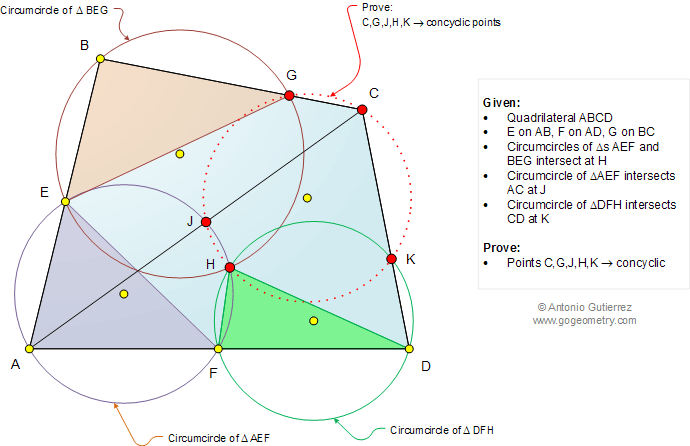Infographic Geometry Problem 1052: Triangle, Quadrilateral, Three Circumcircles, Circle, Concyclic Points, Cyclic Quadrilateral. Level: High School, SAT Prep, College, Mathematics Education

< PREVIOUS PROBLEM  |  NEXT PROBLEM >

 The figure below shows a quadrilateral ABCD with a point E on AB, F on AD, and G on BC so that the circumcircles of triangles AEF and BEG intersect at H. The circumcircle of triangle AEF intersects AC at J and the circumcircle of triangle DFH intersects CD at K. Prove that C, G, J, H, and K are concyclic points.Home | Search | Geometry | Problems | All Problems | Open Problems | Visual Index | 10 Problems | 1051-1060 | Triangle | Quadrilateral | Circumcircle | Circle | Cyclic Quadrilateral | Concyclic Points | Email | Solution / comment | by Antonio Gutierrez Last updated: Oct 30, 2014